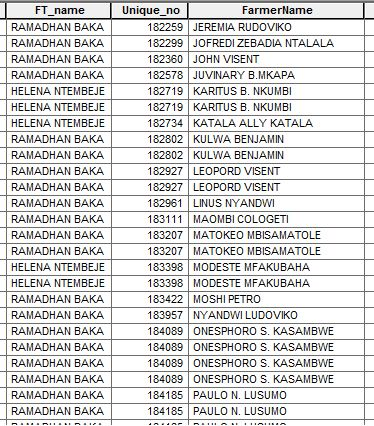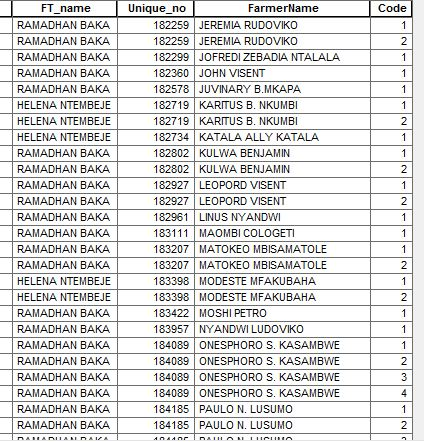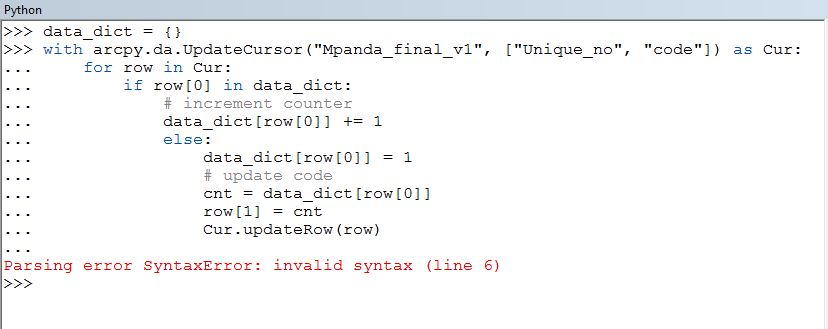# Calculating counting field

1611
5
03-07-2017 10:02 PMOccasional Contributor

Hello guys,

I have a table below;From there i created a new field "code" so i want to calculate a sequential numbers based on how much the "Unique_no" appears like the one below;Regards,

Kelvin.

1 Solution

Accepted SolutionsbyMVP Frequent Contributor

You could do this with an update cursor and a dictionary, something like this...

``````data_dict = {}
with arcpy.da.UpdateCursor(fcName, ["Unique_no", "Code"]) as Cur:
for row in Cur:
if row in data_dict:
# increment counter
data_dict[row] += 1
else:
data_dict[row] = 1
# update code
cnt = dat_dict[row]
row = cnt
Cur.updateRow(row)
‍‍‍‍‍‍‍‍‍‍‍‍``````
5 RepliesbyMVP Frequent Contributor

You could do this with an update cursor and a dictionary, something like this...

``````data_dict = {}
with arcpy.da.UpdateCursor(fcName, ["Unique_no", "Code"]) as Cur:
for row in Cur:
if row in data_dict:
# increment counter
data_dict[row] += 1
else:
data_dict[row] = 1
# update code
cnt = dat_dict[row]
row = cnt
Cur.updateRow(row)
‍‍‍‍‍‍‍‍‍‍‍‍``````Occasional Contributor

Thanks Neil,

See if I'm wrong somewhere because i am not good in python.byMVP Frequent Contributor

Firstly try and run this as a script in IDLE or pyscripter or something.

You have the indentation wrong. The line starting else:

Should be at the same level as the if:

Indentation in python is a crucial part of the language syntax.

Try doing a bit of reading up on python first.byMVP Frequent Contributor

And the last 3 lines also at the same indentation level as the if:byMVP Esteemed Contributor

Using defaultdict allows the code to be simplified/condense a bit:

``````from collections import defaultdict

data_dict = defaultdict(int)
with arcpy.da.UpdateCursor(fcName, ["Unique_no", "Code"]) as cur:
for uniq, code in cur:
data_dict[uniq] += 1
cur.updateRow([uniq, data_dict[uniq]])‍‍‍‍‍‍‍‍‍‍‍‍‍``````